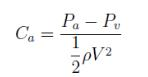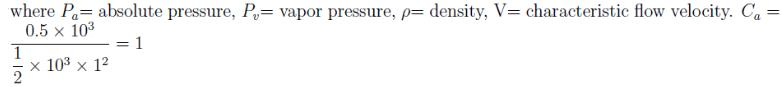Courses

# Test: Vapor Pressure

## 8 Questions MCQ Test Fluid Mechanics | Test: Vapor Pressure

Description
This mock test of Test: Vapor Pressure for Civil Engineering (CE) helps you for every Civil Engineering (CE) entrance exam. This contains 8 Multiple Choice Questions for Civil Engineering (CE) Test: Vapor Pressure (mcq) to study with solutions a complete question bank. The solved questions answers in this Test: Vapor Pressure quiz give you a good mix of easy questions and tough questions. Civil Engineering (CE) students definitely take this Test: Vapor Pressure exercise for a better result in the exam. You can find other Test: Vapor Pressure extra questions, long questions & short questions for Civil Engineering (CE) on EduRev as well by searching above.
QUESTION: 1

### Which of the following statement is true about vapor pressure of a liquid?

Solution:

Explanation: The vapor pressure of a liquid at a given temperature is given by the pressure ex-erted by the saturated vapor on the liquid surface. When the vapor is saturated, an equilibrium exists between the liquid and the vapor phases. The number of molecules leaving the liquid surface is equal to the number of molecules entering the liquid surface. Hence, it is obvious that vapor pressure will be related to molecular activity and consequently to temperature. With the increase in temperature molecular activity increases as a result of which vapor pressure increases.

QUESTION: 2

### Which of the following option correctly depicts the relation between the vapor pressure of a liquid and it’s temperature?

Solution:

Explanation: Vapor pressure is closely related to molecular activity which is in turn dependant on the temperature of the liquid. With the increase in temperature molecular activity of a vapor increases slowly at first and then rapidly. Similar is the nature of variaion of vapor pressure.

QUESTION: 3

### Which of the following is the condition for the boiling of a liquid?

Solution:

Explanation: As the absolute pressure of a liquid goes below it’s vapor pressure, the formation of vapor bubbles start. Thus, for boiling to start, the absolute pressure of a liquid must be less than or equal to it’s vapor pressure.

QUESTION: 4

Which of the following machines have the possibility of cavitation?

Solution:

Explanation: Cavitation occurs whenever absolute pressure of a liquid drops below it’s vapor pressure. Dropping of pressure is observed mainly in reaction turbines and centrifugal pumps.

QUESTION: 5

The three liquids 1, 2, and 3 with vapor pressures V1, V2 and V3 respectively, are kept under same pressure. If V1 > V2 > V3, which liquid will start boiling early?

Solution:

Explanation: A liquid starts to boil whenever it’s absolute pressure drops below it’s vapor pressure. Thus, the absolute pressure of liquid 1 will drop early, as a result it’ll start boiling early.

QUESTION: 6

Equal amount of a particular liquid is poured into three similar containers, namely 1, 2 and 3, at a temperature of T1, T2 and T3 respectively. If T1 < T2 < T3, the liquid in which container will have the highest vapor pressure?

Solution:

Explanation: Higher the temperature, higher is the molecular activity and consequently, higher is the vapor pressure of a given liquid. Since, container 3 is at the highest temperature, liquid in it will have the highest vapor pressure.

QUESTION: 7

The absolute pressure of a water is 0.5kN above it’s vapor pressure. If it flows with a velocity of 1m/s, what will be the value of Cavitation Number describing the flow induced boiling?

Solution:QUESTION: 8

Which of the following is correct regarding the formation and collapse of vapor bubbles in a liquid?

Solution:

Explanation: Whenever the absolute pressure of a fluid drops below it’s vapor pressure, bubble formation starts. Again, when the fluid pressure goes above the bubble pressure, it’ll collapse. This is how cavitation formation takes place.